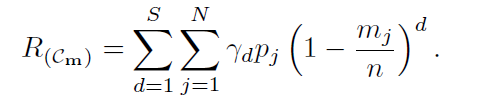# Writing an expression to a power of vector

Hi , I want to write this objective function but I have a problem with writing d as it should be a vector from 1 to S and q is the vector I optimize
This is the expression and q represent m/n\sum^S_{d=1} \sum^N_{j=1} \gamma_d * P_j *(1 - q)^d

The optimization variable is q = m_j / n and all the other variables are inputs

M = 100 ; N = 5 ; S = 10; P_j  = [ .5 ,  .1 ,  .2 , .3 , .4 ] , gammad = [.1 : .1: 1]

cvx_begin
variable q(N)
subject to
sum(q) ==  M ;
0 <= q <= 100;
cvx_end


I tried to use this minimize(sum(sum(gammad’Pj (bsxfun(@power , (1 - q), (1:S))’)))) but still give error

You haven’t told us what are decision (optimization) variables vs. input data.

Nevertheless, have you tried pow_p? Its first argument can be a vector or matrix, to which the power is applied elementwise.

I have updated the post.

Try using pow_p with a fixed value of d, and use a for loop to sum over the values of d I’ll let you work out the details. Or I believe you can just use ^ in this manner instead of pow_p.

N = 5
M = 100
S = 10
P_j = [ .5 , .1 , .2 , .3 , .4 ] ,
\gamma_d = [.1 : .1: 1]
cvx_begin
variable q(N)

            for  i = 1 : S

Total(1:length(q),i) = (pow_p( (1 - q ) , i ))' ;

end

minimize (sum(sum ((P_j'*\gamma_d)*Total)))

subject to
sum(q) ==  M ;
0 <= q <= 1;

cvx_end


I tried to write it in this form but the output is NaN and I cannot figure out where is the problem. Can you please help.

Please show clean MATLAB/CVX code not a bastardized mix of MATLAB/CVX and Latex/Mathjax which makes it impossible to know what you ran. Presuming your code \gamma_d is really gamma_d, your objective function is not even dimensionally consistent.

Thanks so much for your response. I wrote the matlab code below. The issue now is that the optimized value q is NaN

N = 5
M = 100;
S = 10;
P = [ .1 .2 .3 .4 .6]

cvx_begin

variable q(N)

        for  i = 1 : S

Total(1:length(q),i) = (pow_p( (1 - q ) , i ))' ;

end


q has 5 elements. Given that each element of q <= 1, the maximum possible value of sum(q) is 5, thereby rendering sum(q) == 100 impossible to achieve,